Know Thyself Would you like to react to this message? Create an account in a few clicks or log in to continue.Know ThyselfNothing in ExcessKnow Thyself :: AGORA :: LYCEUM Share

# Lost Pythagorean Sacred Geometry understanding material nature by mathematicsAuthorMessage
Guest
GuestSubject: Lost Pythagorean Sacred Geometry understanding material nature by mathematicsMon Jan 12, 2015 10:38 amGuest
GuestSubject: Re: Lost Pythagorean Sacred Geometry understanding material nature by mathematicsMon Jan 12, 2015 1:08 pmSlaughtz

Gender :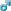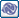Posts : 2593
Join date : 2012-04-28
Age : 31
Location : A stone.Subject: Re: Lost Pythagorean Sacred Geometry understanding material nature by mathematicsFri Mar 03, 2017 2:45 pm [You must be registered and logged in to see this link.]400 units can encompass differing amounts of area.Thin rectangle:175x25=4375In isoceles triangle w/ 36, 36, 108 angles:x+x+x(phi)=400x=110.557Use [You must be registered and logged in to see this link.]Area = ~5,812In the rectangle, 150x50=7,500In equilateral triangle,(√(3)/4)(133.333)^2=7,698In the square,100x100=10,000In a pentagon,(5*80^2) / (4√(5-2√5)) = 11,011In a circle,(400^2) / (4 π) = 12,732Put 1, 1, 36 in the first three fields to represent two sides and the degree. You find the output to be phi.[You must be registered and logged in to see this link.]Last edited by Slaughtz on Fri Mar 03, 2017 8:24 pm; edited 3 times in totalAnfangGender :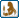Posts : 3902
Join date : 2013-01-23
Age : 39
Location : Castra Alpine GrugSubject: Re: Lost Pythagorean Sacred Geometry understanding material nature by mathematicsFri Mar 03, 2017 4:04 pm The same reason why where it's cold being bulky has its advantages. The more sphere-like the more volume relative to its surface area.Slaughtz

Gender :Posts : 2593
Join date : 2012-04-28
Age : 31
Location : A stone.Subject: Re: Lost Pythagorean Sacred Geometry understanding material nature by mathematicsFri Mar 03, 2017 8:52 pm Reposting From Other Thread (deleting it there):Origins of geometry and primitive proof of quality over quantity. Proportion over size.A 100x100 area is more substantial than a 150x50 area. Also of possible relevance to the phenemonon:Fractal coastlinesHere is Wheeler describing the concept of "middle", proportion, found in ancient Greece; qua the divided line theory and phi. The origin of "hygiene" is symmetry and proportion,  not "cleanliness". The middle of a triangle is not the middle of its largest side. Connects it to water molecule somehow. Quantitative vs Qualitative space. The former splits a triangle down the middle of its longest line. The latter splits the triangle such that each piece maintains the same dimensions/proportion.Slaughtz

Gender :Posts : 2593
Join date : 2012-04-28
Age : 31
Location : A stone.Subject: Re: Lost Pythagorean Sacred Geometry understanding material nature by mathematicsTue Mar 07, 2017 10:46 pm Through my exploration of this, I sought to find the formula for an area of an isosceles triangle, given one only has its perimeter and its internal angles. Specifically so I could prove the proportional relevance of 1/phi.Here is the formula:[You must be registered and logged in to see this link.]a=area, d=vertex angle,p= perimeterNo other fruits yet for my inquiry, except that one may prove the equation for Plato's divided line is x+y+y+z holds true, given the rules of proportional division he specifies. That is, there will always be two parts of same length when one divides a line (point-line, where geometric points are nonexistent absolutes) and the resultant portions all by the same proportions. I demonstrated this with an 18 unit line, which divided into 12/6, then 8/4/4/2. Perhaps one could divide a line in 1/9, then it'd be .1/.9/.9/8.1... Appears it still holds true.Thus a conclusion: Arbitrary divisions, repeated precisely as before (twice more*) equally onto the parts of the first division, begets an orderly pair; a sameness, repetition.*Quantitatively, it is twice more. this involves time. Qualitatively, it is only once, as a pattern, which is there as a proporty(property/qualia,proportion). With regard to Wheeler, 1 is a reification of no-thing. A void, reified into its opposite: every-thing. As a void has sameness and only sameness with the absence of thinginess - and 0 is absurd in representative mathematics (although one could argue 1 is just as absurd in reality), 1 also has only sameness with itself. Using 1 as a representation for the 'emergent' order of repeated and equal division is appropriate. 0 appears incompatible with the human mathematical system, when utilizing divisions. 0/x appropriately always gets 0, and x/1 appropriately always returns the same number x. 1 is a mirror of proporty. The addition and subtraction of 1 is the addition and subtraction of potential for the absorption of proporties.Satyr
DaemonGender :Posts : 31487
Join date : 2009-08-24
Age : 56
Location : HyperboreaSubject: Re: Lost Pythagorean Sacred Geometry understanding material nature by mathematicsSat Oct 01, 2022 8:01 pm [You must be registered and logged in to see this link.]_________________γνῶθι σεαυτόνμηδέν άγαν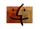FreyjaGender :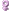Posts : 88
Join date : 2022-09-16
Location : Many moons awaySubject: Re: Lost Pythagorean Sacred Geometry understanding material nature by mathematicsMon Oct 17, 2022 11:03 pm The boy with incredible Brain (Super Genius Documentary)Subject: Re: Lost Pythagorean Sacred Geometry understanding material nature by mathematicsLost Pythagorean Sacred Geometry understanding material nature by mathematicsPage 1 of 1
 Similar topics» DNA & and Sacred
» The Sacred Harp
» Knowledge/Understanding
» Words
» THE LEFT - Understanding 1968

Permissions in this forum:You cannot reply to topics in this forum